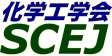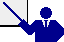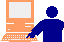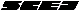English page## \$B9V1i?=\$79~\$_J}K!(B

### Web \$B\$K\$h\$k?=\$79~\$_\$N%5%s%W%k(B

Web \$B?=\$79~\$_\$K4X\$9\$k0lHLE*\$JCm0U;v9`(B\$B!"(B\$B?=9~=q\$N3F9`L\\$K\$D\$\$\$F\$N>\:Y\$J@bL@(B \$B\$H(BWeb \$B?=\$79~\$_%U%)!<%`\$N5-F~Nc(B \$B\$bMQ0U\$7\$F\$\$\$^\$9\$N\$G!"\$4;2>H\$/\$@\$5\$\$!#(B

\$B%*%s%i%\$%s?=\$79~\$_\$N2008\$BG/(B11\$B7n(B6\$BF|\$+\$i(B11\$B7n(B25\$BF|(B22\$B;~\$^\$G(B\$B\$G\$9!#(B

\$B9`L\L>\$O3F9`L\\$N%X%k%W\$K%j%s%/\$7\$F\$\$\$^\$9!#%X%k%WI=<(8e\$3\$N%Z!<%8\$KLa\$k\$H\$=\$l\$^\$G\$NF~NOFbMF\$,>C\$(\$k\$3\$H\$,\$"\$j\$^\$9!#(B

\$BJ,N`HV9f(B \$BH/I=7A<0(B \$B9V1iBjL\(B\$BBh#14uK>!'(B\$B!!!!(B \$BBh#24uK>!'(B\$B!!!!(B \$B"+(B\$BJ,N`HV9f0lMwI=(B\$B\$+\$iA*\$s\$G\$/\$@\$5\$\$!#(B O: \$B8}F,(B P: \$B%]%9%?!<(B \$B@5(B(\$B!&L>M@2q0w(B) \$B3X(B(\$B@82q0w(B) \$BIt(B(\$B2q@52q0w(B) \$B6&(B(\$B:E3X6(2q0w(B) \$BB>(B \$B@5(B(\$B!&L>M@2q0w(B) \$B3X(B(\$B@82q0w(B) \$BIt(B(\$B2q@52q0w(B) \$B6&(B(\$B:E3X6(2q0w(B) \$BB>(B \$B@5(B(\$B!&L>M@2q0w(B) \$B3X(B(\$B@82q0w(B) \$BIt(B(\$B2q@52q0w(B) \$B6&(B(\$B:E3X6(2q0w(B) \$BB>(B \$B@5(B(\$B!&L>M@2q0w(B) \$B3X(B(\$B@82q0w(B) \$BIt(B(\$B2q@52q0w(B) \$B6&(B(\$B:E3X6(2q0w(B) \$BB>(B \$B@5(B(\$B!&L>M@2q0w(B) \$B3X(B(\$B@82q0w(B) \$BIt(B(\$B2q@52q0w(B) \$B6&(B(\$B:E3X6(2q0w(B) \$BB>(B \$B@5(B(\$B!&L>M@2q0w(B) \$B3X(B(\$B@82q0w(B) \$BIt(B(\$B2q@52q0w(B) \$B6&(B(\$B:E3X6(2q0w(B) \$BB>(B \$B@5(B(\$B!&L>M@2q0w(B) \$B3X(B(\$B@82q0w(B) \$BIt(B(\$B2q@52q0w(B) \$B6&(B(\$B:E3X6(2q0w(B) \$BB>(B \$B@5(B(\$B!&L>M@2q0w(B) \$B3X(B(\$B@82q0w(B) \$BIt(B(\$B2q@52q0w(B) \$B6&(B(\$B:E3X6(2q0w(B) \$BB>(B \$B@5(B(\$B!&L>M@2q0w(B) \$B3X(B(\$B@82q0w(B) \$BIt(B(\$B2q@52q0w(B) \$B6&(B(\$B:E3X6(2q0w(B) \$BB>(B \$B@5(B(\$B!&L>M@2q0w(B) \$B3X(B(\$B@82q0w(B) \$BIt(B(\$B2q@52q0w(B) \$B6&(B(\$B:E3X6(2q0w(B) \$BB>(B \$B@5(B(\$B!&L>M@2q0w(B) \$B3X(B(\$B@82q0w(B) \$BIt(B(\$B2q@52q0w(B) \$B6&(B(\$B:E3X6(2q0w(B) \$BB>(B \$B@5(B(\$B!&L>M@2q0w(B) \$B3X(B(\$B@82q0w(B) \$BIt(B(\$B2q@52q0w(B) \$B6&(B(\$B:E3X6(2q0w(B) \$BB>(B \$B@5(B(\$B!&L>M@2q0w(B) \$B3X(B(\$B@82q0w(B) \$BIt(B(\$B2q@52q0w(B) \$B6&(B(\$B:E3X6(2q0w(B) \$BB>(B \$BH/I=\$NA0\$K\$"\$k%\%?%s\$GEPCE \$B2=3X9)3X2q\$G\$O!";f\$N9V1i?=9~=q\$O\$9\$G\$KGQ;_\$5\$l!"(BWeb, E-mail \$B\$G9V1i?=9~\$r3Q1Q?t;z\$G\$*4j\$\$\$7\$^\$9!#9V1iMW;]869F\$NDs=PEy\$KI,MW\$H\$J\$j\$^\$9!#(B\$B"+(B \$BH/I=\$r1Q8l\$GI=5-\$7\$?>l9g\$O!"(B\$B!!(B \$B?=9~ \$B!VHw9M!WMs\$Ol9g\$N\$_5-F~\$7!"DL>o\$O2?\$b5-F~\$7\$J\$\$\$G2<\$5\$\$!#(B

\$B2=3X9)3X2q(B \$BBh(B74\$BG/2q(B(\$B2#IM(B)(C) 2008 (\$B
Most recent update: 2008-12-25 11:06:19
E-mail: inquiry-74awww2.scej.org
This page was generated byeasp 2.26; mknote 2.26a (C)1999-2008 kawase